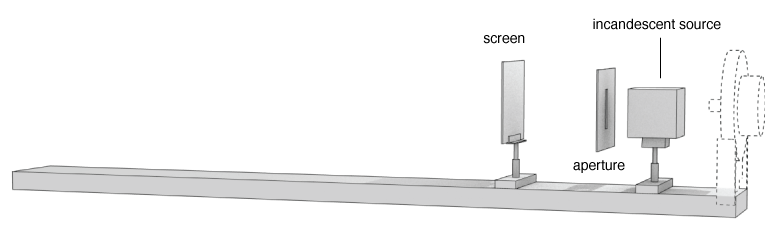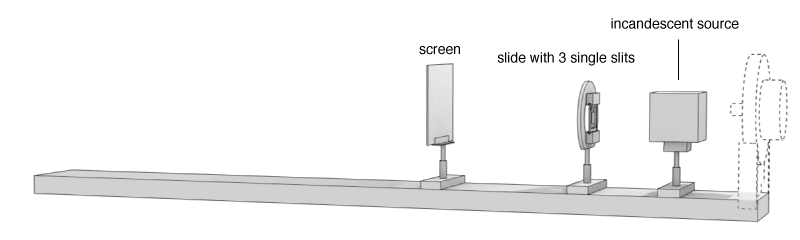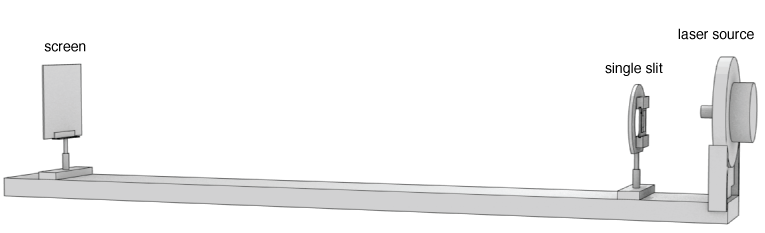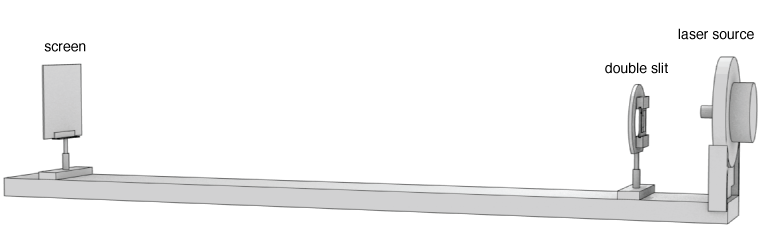## Physics 204 - Lab 6 - Interference of Light

### Names and Such

And the name of the lab instructor present:

# Interference of Light

### Goals

This lab will demonstrate the wave nature of light. You will use this property to calculate properties of light.

### Safety NoticeThis lab uses a very low power laser. All lasers should be handled with care, and you should never look directly into the beam.

### Equipment check

Please make sure your station has all of the following items. If not, check again, then talk to your lab instructor.

1. One optics track
2. One Screen with holder
3. One slide holder
4. Two slides with slits
5. One laser source
6. One incandescent source
7. One index card
8. One pair of scissors
9. One Digital Caliper
10. One Ruler### Intro to Interference of Light

One of the consequences of the wave nature of light is that the principle of superposition applies: two waves can be combined by simply adding their amplitudes. If both waves are at their maximum amplitude $A$, then the result is $2A$. If they are both at the minimum amplitude, $-A$, then the result will be $-2A$. If one is at a maximum, and the other a minimum, then the sum of the two waves at that point will be zero. An easy way to control the relation between two waves is by having them travel different distances before combining, thus creating a path-length difference and allowing for interference effects. This lab will explore the physics behind this idea.

#### Coherent vs. Incoherent Wave sources

Definition: Two sources are considered coherent if they emit waves that maintain a constant phase relation.Effectively, this means that the waves do not shift relative to one another as time passes. In a laser (which is a coherent source) all the waves that are emitted start with the same phase. In an incandescent bulb, the light waves are created due to more random processes, so the light waves emitted start with different phases and the phase relation between different waves will not be constant as time evolves.

If a source of waves is coherent, then we can predict when there will be constructive and destructive interference. The figure at left shows two sources one coherent, the other incoherent.

### Report Question

• In your own words: explain the difference between coherent and incoherent sources? Do some research and find some examples of coherent and incoherent wave sources (they need not be light waves - sound sources or other mechanical waves can also be coherent or incoherent.)

### Large opening and Incoherent Light

Let's begin by examining what happens to regular, non-coherent white light as it passes through an aperture (that's just a technical term for for a hole, or gap). You'll make your own aperture out of a piece of paper. Try to make a rectangular slit about 2-3 millimeters in width in the index card provided, using the scissors.

First, predict what will show up on a screen when light from the incandescent bulb passes through a rectangular opening. Try to describe in detail what will appear on the screen. (Will it be a rectangle the same size as the aperture? Or bigger, smaller? Will it have blurry edges or sharp edges?)

After you have predicted what you would expect to see, then set up the optics track as shown. Use the incandescent light (just a regular lightbulb). You can start with the light source about 20 cm from one end. Then hold your rectangular opening right next to the opening of the incandescent source. The screen can go about 20 cm past the opening. (Leave the laser in place, even though you won't be using it yet.)Now, sketch an intensity profile based on what you see on the screen. Here's an example. Let's say you let the white light pass through a large circular aperture. It might make a shape on the screen like the one shown on the left side of this figure below. If you plotted the intensity of light (how bright it is) at every point along the dotted line, you would obtain something like the plot on the right - an intensity profile. Don't worry about the absolute units of intensity on the vertical axis. We can call the brightest point 1 unit of intensity, and scale the rest to that.### Small slits with Incoherent Light

The next procedure will be to replace the large opening with slits that are very small - closer to the wavelength of the light being used. Before doing this however, make a prediction about what you will see when you replace the wide rectangular gap with a very narrow (~ 150 μm) single slit.

After you make a prediction, obtain the slide with part number 1000885. It should have 3 single slits and 1 double slit. Set up the slide so the 3 single slits are vertically aligned on the bottom of the slide. Place the slide about 10-15 cm away from the incandescent light source. You might need to adjust the heights of the various components, like the screen, the slides, and the light source. This can be done by loosening the thumbscrews and raising or lowering the inserts.

After it is all adjusted, you should be able to see the illumination from the three single slits on the lower section of the viewing screen. Sketch an intensity vs. position graph for a line that passes through each of the three slits. Did you prediction match the result?### Report Question

Include responses to the following questions with your graphs of intensity.

• Are the maximum intensities the same for each slit? Explain why the maximums could be different values.
• Are the widths of the intensity profiles the same? How do they differ?
• Are the edges of the intensity profiles sharp? or smooth? (i.e. are the shadows crisp, or blurry?)

### Monochromatic vs. PolychromaticAnother distinguishing aspect of light sources is the distribution of wavelengths present. An incandescent bulb will emit light with many different wavelengths (i.e. colors). A monochromatic source, like a laser, will emit light of one specific wavelength. Interference effects are harder to observe with polychromatic light.

This lab will use a red laser to generate a coherent, monochromatic source of light. At this point, turn on your laser using the switch on the back. Again, do not look directly into the beam.

### Report Question

Include responses to the following questions with your graphs of intensity.

• Explain the difference between monochromatic and polychromatic light. Give examples of each kind. Why are interference effects harder to observe with polychromatic light? Describe how could you create a monochromatic light source using polychromatic rays from the sun? (hint: Newton was among the first to figure out how to do this)

### A Coherent Source, and a Single Slit

Now we will use the coherent monochromatic light source (a laser) and investigate what happens when it passes through slights with widths comparable to its wavelength. Just like before, make a prediction as to what you will see on the screen when the laser light passes through a small single slit. Sketch an intensity profile of this prediction and describe the prediction below.

After making a prediction, set up the optics track as shown in the figure. Put the screen as far as you can on one end, and the source and the single slit slide holder near to each other on the other end. You'll note the single slit slide has 3 single slits and 1 double slit set. Adjust the laser so it passes through the center slit from the 3 single slits. You may need to adjust the direction of the beam by using the thumbscrews located on the back of the laser module. (Be Gentle!! The laser is delicate and you can easily break it by turning these screws too tightly) Sketch an intensity pattern of the resulting light that hits the screen. You'll notice that the intensity profile looks nothing like the previous profiles for the polychromatic, incoherent light.### A Coherent Source, and a Double Slit

Now, replace the single slit slide with the slide labeled 4 double slits (part number 1000597). The pattern produced by a double slit is somewhat easier to understand. We will use the interference pattern that forms on the screen to calculate the wavelength of the laser light. Set up your optical track as shown.Adjust the thumbscrews on the back of the laser if you need to align the beam. Choose one of the four double slit geometries on the slide. (Hint: the smaller the slit spacing, the easier it will be to see a good interference pattern) You'll want the screen to be as far away from the slits as possible (while still remaining on the track).If you inspect the slide with 4 double slit sets, you'll see a label on one side that contains information about the the slit geometry. There are two important dimensions to consider: the slit width, and the slit spacing. The slit width refers to how wide each individual slit it. On the slide, this has been given the variable $a$. The slit spacing is the distance between the two slides. On the slide, this is labeled as $d$.

The measurement you will perform will be to measure the distance between interference fringes as they appear on the screen. Here is an interference pattern and intensity plot from a typical double slit experiment. You should see something resembling the interference pattern on the screen. Yours will depend on which slit you pick, and how far your slit-screen distance $L$ is.To obtain a relationship between the wavelength of the light, and the interference pattern, only a little geometry is needed. Since constructive interference (which makes bright spots) occurs when the path length difference between the two waves ($r_1$ and $r_2$) is a whole number of wavelengths. we need to satisfy the following: $$\Delta r = m \lambda$$ The figure shows what angle $\lambda$ is as well as the two paths in question.$\Delta r$ can be found be using : $$\frac{\Delta r}{d} = \sin \theta$$ Since the angle $\theta$ is very small (usually less than 10°) we can also say: $$\sin \theta \approx \tan \theta$$ Let's check though: how big is angle theta for the first fringe in this setup?

From the setup, $\tan \theta$ can be calculated using the distance $L$ (measured with the marks on the track) and the distance $y$ (best measured using the digital caliper). That should be enough information to find a relationship between the the fringe spacing (what you are measuring: $y$) and the wavelength of the light (what you are trying to determine based on the measurement: $\lambda$).

What is the wavelength of the light in nanometers?

What is $\lambda$ [nm] = please log in

Take a picture of your interference pattern using your phone. You will need to include it in your report. (If you don't have a camera phone, ask the TA to borrow the lab camera.)

### Report Question

Include responses to the following questions with your graphs of intensity.

• Derive the formula that relates the wavelength of the light to the interference pattern fringe spacing. Show your work with algebra and geometry. Include a picture of your interference pattern as it appears on the screen. Provide an estimate using the measurements you obtained for the wavelength of the laser light.

### Done?

First, check your station for the equipment. Please return the station to a neat and orderly arrangement as shown in this picture. Once you have done that, click the checkbox below.Now, click this button below to view your filled out worksheet. Print it out and get it signed by your lab instructor before leaving.